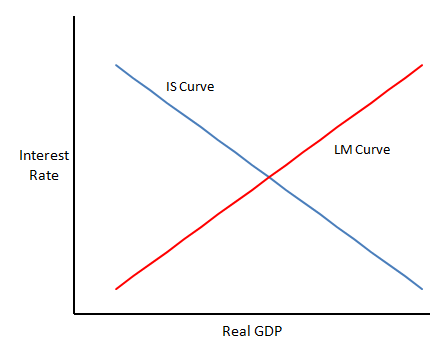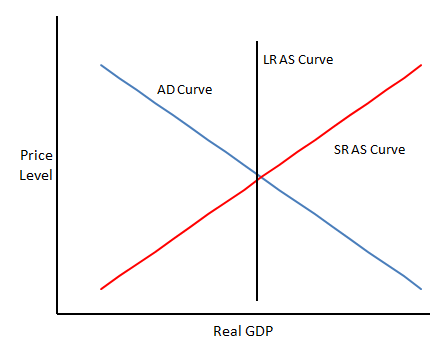Friday, 3 January 2014

IS/LM, AS/AD and the Exchange Rate

Lars Christensen has a post in which he uses an Aggregate Supply / Aggregate Demand (AS/AD) diagram to talk about monetary offset in the eurozone.  This is the idea that if the central bank is pursuing an inflation target, then fiscal policy can have no effect because it will be automatically be countered by monetary policy.  A good description of monetary offset is here.

This reminded me of one of the things that puzzled me when I first learned about AS/AD and its interaction with IS/LM.

In the standard IS/LM model, the IS curve and the LM curve each describe a different relationship between the interest rate and real GDP.  Each assumes that certain other variables are fixed, including the general price level.  Thus for any given price level, we can deduce a level of real GDP from where the IS curve meets the LM curve.  This also determines the interest rate.The relationship between the price level and real GDP gives us our aggregate demand curve.  This is usually assumed to be downward sloping, so that as the price level falls, GDP rises (although it has been argued that it might be upwards sloping in certain circumstances).

To determine where we are on the aggregate demand curve, we combine it with an aggregate supply curve.  Here, the convention is to draw an aggregate supply curve that slopes upwards in the short run, but is vertical in the long run.  The idea is that price stickiness in the short run limits price rises so that changes in demand create real changes in output, but in the long run, once prices have fully adjusted, output must return to its equilibrium level.  (AS/AD is sometimes done by references to changes, rather than absolute levels, i.e. as inflation against real GDP growth).So far, this is all pretty standard.  There's plenty of stuff there to question the validity of, but the issue I want to look at is to do with the exchange rate.

The first thing to note is that the position of the short run AS curve depends on the exchange rate.  A depreciation of the exchange rate will increase the effective price of foreign goods.  This will tend to increase the price of domestic goods that compete with foreign goods: exports or domestic substitutes for imports.  At the same time, higher import costs will potentially reduce real wages.  To the extent that there is also real wage stickiness, nominal wages will rise by more than they otherwise would.  So, a depreciation will move the short run AS curve upwards.

Again, this should not be controversial and I have see it discussed before, but it's often not mentioned.

The interesting bit comes though when we try to combine this with what is going on with IS/LM.  The issue here is that one of factors influencing the exchange rate is the interest rate.  We already know that a change in either the IS curve or the LM curve will shift the AD curve.  However, because it also has an impact on the interest rate we can now see that it will also move the short run AS curve.

This seems to me to be fairly obvious.  I learned this stuff in the 80s and I'd say, in fact, that it's quite hard to explain what happened to the UK economy in the early 80s without taking these things into account.  But surprisingly, it was never discussed, even with the derivations of IS/LM (Mundell-Fleming and IS/LM/BP) that are supposed to deal with the open economy.  Yet it impacts on the validity of the results of those models.

How does this relate to monetary offset?  Well the point is that we can no longer assume a fixed AS curve when considering the impact of changes in the balance of fiscal and monetary policy.  If monetary policy is tightened in response to expansionary fiscal policy, we would expect the exchange rate to appreciate and for the short run AS curve to move outwards.  So even if the result is to leave the AD curve where it is, there is no way for monetary offset to leave both inflation and growth unchanged.

I should say that this is as much about flaws in the whole IS/LM framework as it is about monetary offset.  Trying to extend the framework to incorporate this feedback gets very messy as it turns out that the position of the whole AS curve depends on where it crosses the AD curve.  It's one of the reasons I gave up on IS/LM.